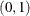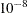### PROC SIMNORMAL Statement

`PROC SIMNORMAL` DATA=SAS-data-set <options> ;

The PROC SIMNORMAL statement invokes the SIMNORMAL procedure. Table 86.1 summarizes the options available in the PROC SIMNORMAL statement.

Table 86.1: Summary of PROC SIMNORMAL Statement Options

Option

Description

Specify Input and Output Data Sets

Specifies input data set (TYPE=CORR, COV, and so on)

Creates output data set that contains simulated values

Seed Values

Specifies seed value (integer)

Requests reinitialization of seed for each BY group

Control Contents of OUT= Data Set

Requests seed values written to OUT= data set

Requests conditioning variable values written to OUT=data set

Control Number of Simulated Values

Specifies the number of realizations for each BY group written to the OUT= data set

Singularity Criteria

Sets the singularity criterion for Cholesky decomposition

Sets the singularity criterion for covariance matrix sweeping

The following options can be used with the PROC SIMNORMAL statement.

DATA=SAS-data-set

specifies the input data set that must be a specially structured TYPE=CORR, COV, UCORR, UCOV, or SSCP SAS data set. If the DATA= option is omitted, the most recently created SAS data set is used.

SEED=seed-value

specifies the seed to use for the random number generator. If the SEED= value is omitted, the system clock is used. If the system clock is used, a note is written to the log; the note gives the seed value based on the system clock. In addition, the random seed stream is copied to the OUT= data set if the OUTSEED option is specified.

SEEDBY

specifies that the seed stream be reinitialized for each BY group. By default, a single random stream is used over all BY groups. If you specify SEEDBY, the random stream starts again at the initial seed value. This initial value is from the SEED= value that you specify. If you do not specify a SEED=value, the system clock generates this initial seed.

For example, suppose you had a TYPE=CORR data set with BY groups, and the mean, variances, and covariance or correlation values were identical for each BY group. Then if you specified SEEDBY, the simulated values in each BY group in the OUT= data set would be identical.

OUT=SAS-data-set

specifies a SAS data set in which to store the simulated values for the VAR variables. If you omit the OUT=option, the output data set is created and given a default name by using the `DATAn` convention.

See the section OUT= Output Data Set for details.

NUMREAL=n

specifies the number of realizations to generate. A value of NUMREAL=500 generates 500 observations in the OUT=dataset, or 500 observations within each BY group if a BY statement is given.

NUMREAL can be abbreviated as NUMR or NR.

OUTSEED

requests that the seed values be included in the OUT= data set. The variable `Seed` is added to the OUT= data set. The first value of `Seed` is the SEED= value specified in the PROC SIMNORMAL statement (or obtained from the system clock); subsequent values are produced by the random number generator.

OUTCOND

requests that the values of the conditioning variables be included in the OUT= data set. These values are constant for the data set or within a BY group. Note that specifying OUTCOND can greatly increase the size of the OUT= data set. This increase depends on the number of conditioning variables.

SINGULAR1=number

specifies the first singularity criterion, which is applied to the Cholesky decomposition of the covariance matrix. The SINGULAR1= value must be in the range. The default value is. SINGULAR1 can be abbreviated SING1.

SINGULAR2=number

specifies the second singularity criterion, which is applied to the sweeping of the covariance or correlation matrix to obtain the conditional covariance. The SINGULAR2=option is applicable only when a CONDITION statement is given. The SINGULAR2= value must be in the range. The default value is. SINGULAR2 can be abbreviated SING2.